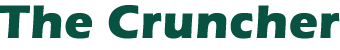6Advanced TechniquesMany longer programs could benefit from this memory-saving technique, which saved 7,000 bytes in the music DATA within the author's music program.

Programs are written every day using DATA statements. Often the numbers in these statements are SOUND and PLOT commands and happen to be in range of 0 to 255. Frequently, the program loads these numbers a matrix. This method of DATA storage is inefficient; it wastes lots of memory.

There is, however, a way to solve this problem, and an easy way to change already existing programs to a more compact form. Using "Cruncher," I knocked 7K — that's right, 7000 bytes — off a program. It took about 40 minutes, and that includes debugging. Many programs can easily be done in half that time.

Each character on the Atari has an ATASCII value ranging from 0 to 255. Look in your BASIC Reference Manual, Appendix C. Take, example, the letter A. Its corresponding number is 65. By using we can convert number (using one to three digits) to a single character using only one character. It would be a very process if we took number, looked it up on the chart, then replaced the number in a program with a single character.

That's where the Cruncher comes. It won't do all of the work, but it will do most of it. We can further save memory by condensing of these single characters into one large string instead of a matrix. This is the big memory saver: each character in a matrix takes about seven bytes, but a string takes only one. So, pull out a program with a lot of numbers and let's get to work. (Note: This is not a standard procedure. Your program may require modifications of the process of conversion. Read through the procedure and think about what you are doing; otherwise, you may find yourself hopelessly lost.)

First, type the following subroutine into your Atari, and LIST it to cassette or disk. This way you can load it on top of program to be converted.

```0 A=PEEK(136)+PEEK(137)*256:? "WHAT LINE";:INPUT X:TRAP 32003:GOTO 32000
32000 LI=PEEK(A)+PEEK(A+1)*256:IF LI<>X THEN A=A+PEEK(A+2):GOTO 32000
32001 A=A+1:IF PEEK(A)=90 THEN READ D:POKE A,D
32002 GOTO 32001
32003 END
```

Second, load the program to be converted. Put in a DIM statement and DIMension a string, say A\$, to the number of numbers in the DATA statements. If your program READs the DATA and then puts it in a matrix, get rid of the READ statements. Otherwise, change a routine like this:

`100 FOR I=1 TO 100:READ A,B:PLOT A,B:NEXT I`

to this:

`100 FOR I=1 TO 100:A=ASC(A\$(I,I)):B=ASC(A\$(I+1,I+1):PLOT A,B:NEXT I`

To be fully funcitonal with the original statement, this should actually read:
`100 FOR I=1 TO 100 STEP 2:A=ASC(A\$(I,I)):B=ASC(A\$(I+1,I+1):PLOT A,B:NEXT I`

or better yet:

`100 FOR I=1 TO 100:PLOT ASC(A\$(I,I)),ASC(A\$(I+1,I+1)):NEXT I`

To be fully funcitonal with the original statement, this should actually read:
`100 FOR I=1 TO 100 STEP 2:PLOT ASC(A\$(I,I)),ASC(A\$(I+1,I+1)):NEXT I`

If your program handles the DATA in a different way, then it's up to you to figure out the rest of that part on your own.

Now we are almost ready to convert the DATA. Before we can put the characters into A\$, we must have an A\$. It is already DIMensioned, but we must add space for the characters in the program. Get an idea as to approximately how many numbers are to be converted, say 200. Then type something like this into your program:

```50 A\$(1,50)="ZZZZZZZZZZZZZZZZZZZZZZZZZZZZZZZZZZZZZZZZZZZZZZZZZZ"
52 A\$(51,100)="ZZZZZZZZZZZZZZZZZZZZZZZZZZZZZZZZZZZZZZZZZZZZZZZZZZ"
54 A\$(101,150)="ZZZZZZZZZZZZZZZZZZZZZZZZZZZZZZZZZZZZZZZZZZZZZZZZZZ"
56 A\$(151,200)="ZZZZZZZZZZZZZZZZZZZZZZZZZZZZZZZZZZZZZZZZZZZZZZZZZZ"
58 A\$(201,225)="ZZZZZZZZZZZZZZZZZZZZZZZZZ"```

It doesn't hurt to put in some extras; you can always take them out later. To easily duplicate a line, just type it, press RETURN, move the cursor back to the line number, change it, and press RETURN. (Note: You must use capital Z's.) Once you have done this, type RUN. Tell the computer what line your Z's start at (in our sample, 50). Now, wait while the computer figures everything out. When READY appears, LIST the program and see what happens. Voilá! The Z's now look like a lot of garbage!

Fourth, and last, get rid of any extra Z's and delete line 0, lines 32000 to 32003, and all of the numerical DATA statements. Now type RUN and watch your program run faster than ever. Sit back and say to yourself, "Gee, that was easy. What program should I fix next?"

The Mystery Revealed

For those of you who would like to know how this program works, I will explain it step by step. The first thing the computer does is find out where the program is stored in RAM. By PEEKing addresses 136 and 137, the Cruncher finds out the first address of the program. The TRAP is so that when the computer is out of DATA, it ENDs without an error.

Next, the computer finds line X. The first three bytes of each line give very important information. The first two tell the line number, and the third tells the length. To check if we are at line X, we first find out at which line we are. If LI isn't equal to X, we must advance the pointer to the next line. We do this by adding the length of the line to our original number and trying again.

Now the conversion process begins. A loop begins that checks each address to see if it is 90, or a Z. If it is, the program READs a piece of DATA and POKEs it into the program. We then loop back and continue the process. When we run out of DATA, the TRAP is sounded and the program ENDs.

Listing. The Cruncher.